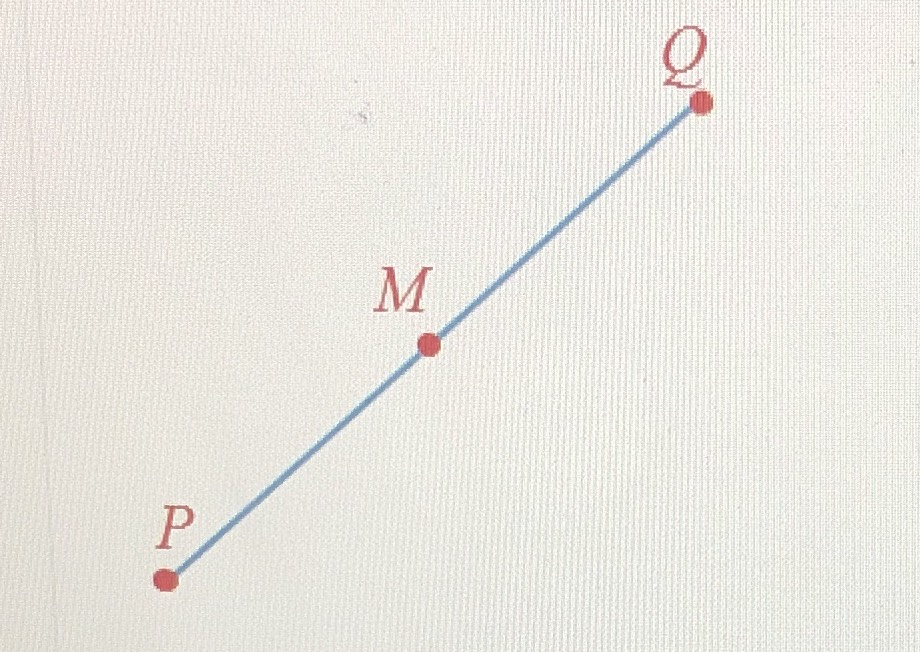¿Todavía tienes preguntas de matemáticas?

Pregunte a nuestros tutores expertos
Algebra
QuestionPoint $$\mathrm{M}$$ is the midpoint of $$\mathrm{PQ}$$. If $$\mathrm{PM}=25$$, and $$\mathrm{MQ}=5x+5$$, determine the value of $$x$$.

$$x=4$$